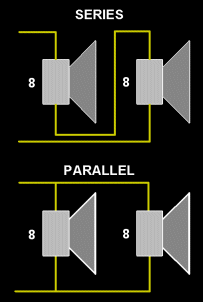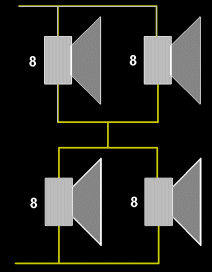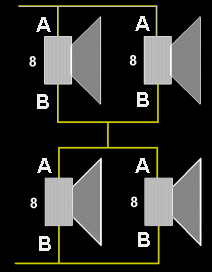# What Do Speaker Impedances Mean?

### (C) 2004 Hank Wallace

We all know that speakers have a few different ratings: Impedance, power handling, size, frequency response. All these are intuitively simple except for impedance. What does it mean?

The impedance tells you basically how much current will flow through a speaker at a certain voltage. So, ten volts of signal from your amplifier might produce two amps of current through the speaker. Double the voltage to twenty volts and the current will double as well. You’ll get four times the power because the power is voltage times current (2 x 2 = 4).

A lower impedance speaker will accept more power. For example, a 4 ohm speaker will extract more power from your amplifier than a 8 ohm speaker, about twice as much.

The problem most people have with speaker impedances is how to combine speakers safely without blowing the amplifier or speakers. Here’s how.There are two ways to connect speakers, series and parallel. See the picture.

For connecting speakers in series, the impedances always add. For example, the impedance of the series connection pictured is 16 ohms.

For connecting a number of speakers with the same impedance in parallel, the total is the impedance of one speaker divided by the number of speakers. For example, the impedance of the parallel connection pictured is 4 ohms (8 divided by 2).

For two speakers of any impedance connected in parallel, use this formula to compute the total impedance:

Rt = (R1 * R2) / (R1 + R2)Looks complicated, but it’s not. Some examples follow. All numbers are in ohms.

• Let R1 = 8, R2 = 4, then Rt = (8 * 4) / (8 + 4) = 32 / 12 = 2.7 ohms
• Let R1 = 16, R2 = 8, then Rt = (16 * 8) / (16 + 8) = 128 / 24 = 5.3 ohms
• Let R1 = 16, R2 = 4, then Rt = (16 * 4) / (16 + 4) = 64 / 20 = 3.2 ohms

Notice that the total for two speakers in parallel is always less than either speaker. That’s why you have to be careful paralleling speakers. You can quickly arrive at an impedance that is too low for your amp to safely drive.

### Dealing with More Speakers

If you have some speakers in parallel and some in series, you can break the problem up into parts. Work out the parallel impedance first, then add the impedance of the series speaker(s).

For example, you have two 8 ohm and one 4 ohm speakers, and your amp is rated for loads of 4 to 16 ohms. What to do? Let’s try a couple cases.

Paralleling the two 8 ohm speakers gives 4 ohms. Adding the other 4 ohms speaker (connected in series) results in 4 + 4 = 8 ohms total. Good for your amp.Alternatively, you could parallel one 4 and one 8, giving 2.7 ohms (from an example above). Then series connect the other 8 and you have 2.7 + 8 = 10.7 ohms. Also good for your amp.

What about paralleling all three speakers? The two 8’s in parallel give 4 ohms. That in parallel with the other 4 results in a 2 ohm total, bad for your amplifier.

With a pocket calculator and the back of an envelope, you can figure out how to connect the speakers in your cabinets.

For quad speaker cabinets, if all speakers are the same impedance (say, 8 ohms), you can connect two sets in parallel, then connect those sets in series to arrive at the impedance you started with (8 ohms in this example). See the picture.

### Power Ratings

There’s a catch when connecting multiple speakers. If you have two speakers in series, the higher impedance speaker takes more power. For example, for an 8 ohm and 4 ohm speaker in series, the power shared is proportional to the resistance, so the 8 ohm speaker absorbs twice the power of the 4 ohm speaker.

If you have two speakers in parallel, the higher impedance speaker takes less power. For example, for an 8 ohm and 4 ohm speaker in parallel, the power shared is inversely proportional to the resistance, so the 8 ohm speaker absorbs half the power of the 4 ohm speaker.

You have to keep this in mind, because if the two speakers above have the same power rating (50 watts for example), one of them will hog much of the power, making the combination’s power rating not 100 watts, putting one speaker at risk of failure.

### Impedance vs. Quality

Some readers have inquired about the quality of a speaker and how it relates to impedance. The short answer is: Not at all. Many high quality speakers are made every day over a range of impedances. The speaker impedance only indicates how to drive the speaker to full power, that is, use an amplifier with a matching output impedance.

### Speaker Phasing

Do you know that speakers are polarized? They have some marking indicating polarity (+/-, paint dot, red/black, etc.), and that controls how they should be connected. Why?

When current flows into a speaker, it traces a loop through the speaker voice coil, then out the other terminal. As it moves through the voice coil, the coil moves the speaker cone, prompting your mother to run screaming from the house. But these currents are alternating currents, and they flow into and out of the speaker continuously.It turns out that current flowing into terminal ‘A’ (just to pick a name) causes the cone to move OUT. Current flowing into terminal ‘B’ causes the cone to move IN. This determines the polarity of the speaker. These names are arbitrary, and what’s important is matching the polarity of one speaker to another.

When you connect speakers in parallel, you should connect the ‘A’ terminals together, and connect the ‘B’ terminals together. This results in one speaker which has both cones moving in the same direction for the same poalrity current.

When you connect speakers in series, the common connection between the speakers should connect ‘A’ to ‘B’. Then there are left over ‘A’ and ‘B’ terminals for connection to your amplifier. Thus, the same current flowing through both speakers (and it is the same current since they are connected in series) produces the same direction of cone movement.

If you connect the speakers out of phase, you will likely not damage the speakers, but the cabinet’s bass response will be poor.